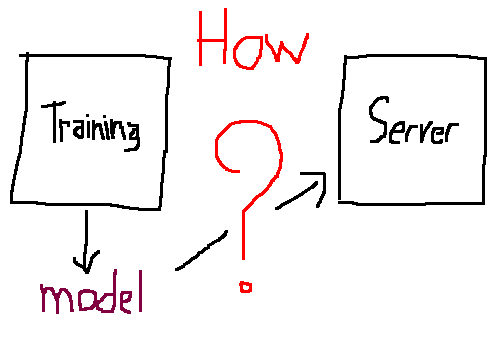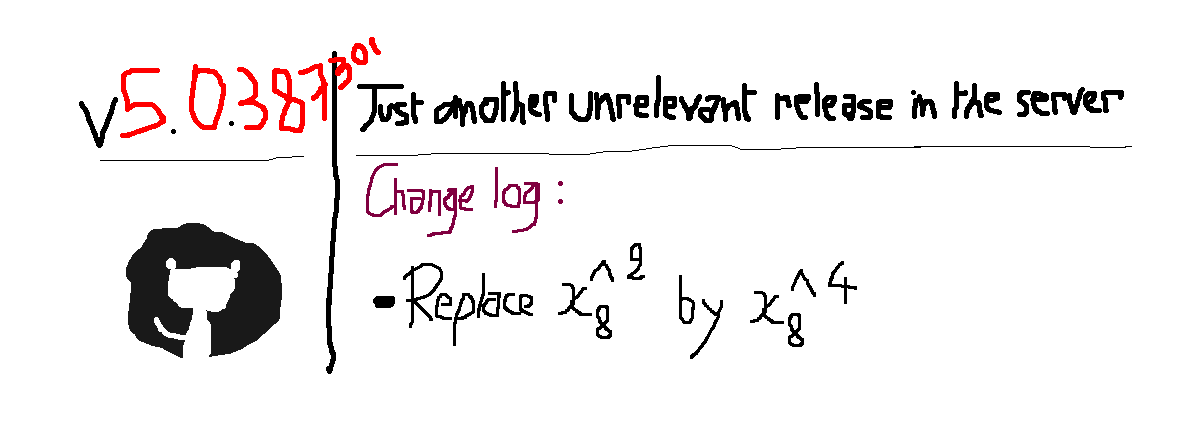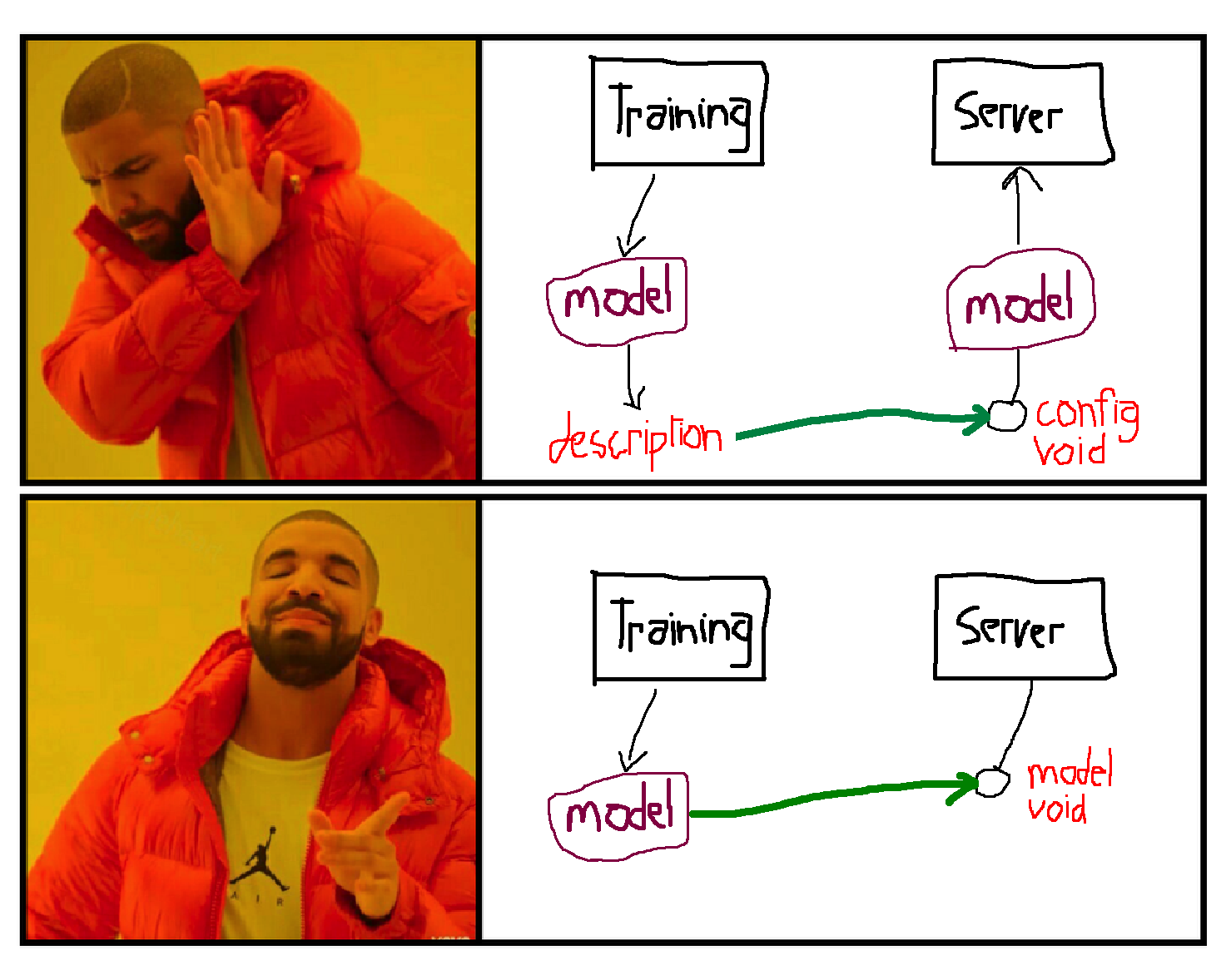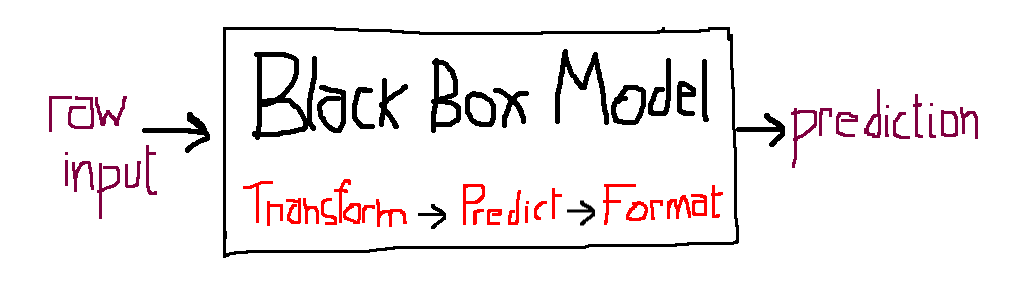## From trained models to prediction servers

After days and nights of hard work, going from feature engineering to cross validation, you finally managed to reach the prediction score that you wanted. Is it over? Well, since you did a great job, you decided to create a microservice that is capable of making predictions on demand based on your trained model. Let’s figure out how to do it. This article will discuss different options and then will present the solution that we adopted at Contentsquare to build an architecture for a prediction server.

## What you should avoid doing

Assuming you have a project where you do your model training, you could think of adding a server layer in the same project. Training models and serving real-time prediction are extremely different tasks and hence should be handled by separate components. I also think that having to load all the server requirements, when you just want to tweak your model isn’t really convenient and — vice versa — having to deploy all your training code on the server side which will never be used is useless.

Thus, a better approach would be to separate the training from the server. This way, you can do all the data science stuff on your local machine or your training cluster, and once you have your awesome model, you can transfer it to the server to make live predictions.So, how could we achieve this?

Frankly, there are many options. I will try to present some of them and then present the solution that we adopted at Contentsquare when we designed the architecture for the automatic zone recognition algorithm.

If you are only interested in the retained solution, you may just skip to the last part.

## What you could do

Our reference example will be a logistic regression on the classic Pima Indians Diabetes Dataset which has 8 numeric features and a binary label. The following Python code gives us train and test sets.

``````from pandas import read_csv
from sklearn.model_selection import train_test_split

url = "https://raw.githubusercontent.com/baatout/ml-in-prod/master/pima-indians-diabetes.csv"
features = ['preg', 'plas', 'pres', 'skin', 'test', 'mass', 'pedi', 'age']
label = 'label'
dataframe = read_csv(url, names=features + [label])
X = dataframe[features]
Y = dataframe[label]
X_train, X_test, Y_train, Y_test = train_test_split(X, Y, test_size=0.33, random_state=42)
``````

### 1. Model coefficients transfer approach

After we split the data we can train our LogReg and save its coefficients in a JSON file.

``````# with X_train, X_test, Y_train, Y_test
from sklearn.linear_model import LogisticRegression

clf = LogisticRegression()
clf.fit(X_train, Y_train)
print(clf.score(X_test, Y_test))

import json
with open('logreg_coefs', 'w') as f:
json.dump(clf.coef_.tolist(), f)
``````

Once we have our coefficients in a safe place, we can reproduce our model in any language or framework we like. Concretely we can write these coefficients in the server configuration files. This way, when the server starts, it will initialize the logreg model with the proper weights from the config. Hurray !
The big advantage here is that the training and the server part are totally independent regarding the programming language and the library requirements.

However, one issue that is often neglected is the feature engineering — or more accurately: the dark side of machine learning. In general you rarely train a model directly on raw data, there is always some preprocessing that should be done before that. It could be anything from standardisation or PCA to all sorts of exotic transformations.
So if you choose to code the preprocessing part in the server side too, note that every little change you make in the training should be duplicated in the server — meaning a new release for both sides. So if you’re always trying to improve the score by tweaking the feature engineering part, be prepared for the double load of work and plenty of redundancy.

Moreover, I don’t know about you, but making a new release of the server while nothing changed in its core implementation really gets on my nerves. I mean, I’m all in for having as much releases as needed in the training part or in the way the models are versioned, but not in the server part, because even when the model changes, the server still works in the same way design-wise.The 387301st release of a prediction server (yeah, I’m exaggerating) due to a simple change in the
feature engineering which doesn’t impact how the server works. Not good.

### 2. PMML approach

Another solution is to use a library or a standard that lets you describe your model along with the preprocessing steps. In fact there is PMML which is a standardisation for ML pipeline description based on an XML format. It provides a way to describe predictive models along with data transformation. Let’s try it !

``````# with X_train, X_test, Y_train, Y_test
import numpy as np
from sklearn_pandas import DataFrameMapper
from sklearn2pmml import PMMLPipeline, sklearn2pmml
from sklearn.linear_model import LogisticRegression
from sklearn.preprocessing import FunctionTransformer

clf = PMMLPipeline([
("mapper", DataFrameMapper([
(['mass'], FunctionTransformer(np.log1p)),
(features, None)
])),
("classifier", LogisticRegression())
])

clf.fit(X_train, Y_train)
print(clf.score(X_test, Y_test))

sklearn2pmml(clf, "Model.pmml", with_repr=True)
``````

So in this example we used sklearn2pmml to export the model and we applied a logarithmic transformation to the “mass” feature. The output file is the following:

``````<?xml version="1.0" encoding="UTF-8" standalone="yes"?>
<PMML xmlns="http://www.dmg.org/PMML-4_3" xmlns:data="http://jpmml.org/jpmml-model/InlineTable" version="4.3">
<Application name="JPMML-SkLearn" version="1.5.6"/>
<Timestamp>2018-09-08T11:13:03Z</Timestamp>
<Extension>PMMLPipeline(steps=[('mapper', DataFrameMapper(default=False, df_out=False,
features=[(['mass'], FunctionTransformer(accept_sparse=False, func=&lt;ufunc 'log1p'&gt;,
inv_kw_args=None, inverse_func=None, kw_args=None,
pass_y='deprecated', validate=True)), (['preg', 'plas', 'pres', 'skin', 'test', 'mass', 'pedi', 'age'], None)],
input_df=False, sparse=False)),
('classifier', LogisticRegression(C=1.0, class_weight=None, dual=False, fit_intercept=True,
intercept_scaling=1, max_iter=100, multi_class='ovr', n_jobs=1,
penalty='l2', random_state=None, solver='liblinear', tol=0.0001,
verbose=0, warm_start=False))])</Extension>
<DataField name="label" optype="categorical" dataType="integer">
<Value value="0"/>
<Value value="1"/>
</DataField>
<DataField name="mass" optype="continuous" dataType="double"/>
<DataField name="preg" optype="continuous" dataType="double"/>
<DataField name="plas" optype="continuous" dataType="double"/>
<DataField name="pres" optype="continuous" dataType="double"/>
<DataField name="skin" optype="continuous" dataType="double"/>
<DataField name="test" optype="continuous" dataType="double"/>
<DataField name="pedi" optype="continuous" dataType="double"/>
<DataField name="age" optype="continuous" dataType="double"/>
<TransformationDictionary>
<DerivedField name="log1p(mass)" optype="continuous" dataType="double">
<Apply function="x-ln1p">
<FieldRef field="mass"/>
</Apply>
</DerivedField>
</TransformationDictionary>
<RegressionModel functionName="classification" normalizationMethod="logit">
<MiningSchema>
<MiningField name="label" usageType="target"/>
<MiningField name="mass"/>
<MiningField name="preg"/>
<MiningField name="plas"/>
<MiningField name="pres"/>
<MiningField name="skin"/>
<MiningField name="test"/>
<MiningField name="pedi"/>
<MiningField name="age"/>
</MiningSchema>
<Output>
<OutputField name="probability(0)" optype="continuous" dataType="double" feature="probability" value="0"/>
<OutputField name="probability(1)" optype="continuous" dataType="double" feature="probability" value="1"/>
</Output>
<RegressionTable intercept="-3.3259427586269217" targetCategory="1">
<NumericPredictor name="log1p(mass)" coefficient="-1.843339687128191"/>
<NumericPredictor name="preg" coefficient="0.06489477494235751"/>
<NumericPredictor name="plas" coefficient="0.029913486211846644"/>
<NumericPredictor name="pres" coefficient="-0.014330833443219983"/>
<NumericPredictor name="skin" coefficient="-0.0035644630076708873"/>
<NumericPredictor name="test" coefficient="-3.9194089727002555E-4"/>
<NumericPredictor name="mass" coefficient="0.16331077682887302"/>
<NumericPredictor name="pedi" coefficient="0.16365918512828412"/>
<NumericPredictor name="age" coefficient="0.02584172148238294"/>
</RegressionTable>
<RegressionTable intercept="0.0" targetCategory="0"/>
</RegressionModel>
</PMML>
``````

Even if PMML doesn’t support all the available ML models, it is still a nice attempt in order to tackle this problem [check PMML official reference for more information]. However, if you choose to work with PMML note that it also lacks the support of many custom transformations. Let’s try another example but this time with a custom transformation `is_adult` on the “age” feature.

``````# with X_train, X_test, Y_train, Y_test
from sklearn_pandas import DataFrameMapper
from sklearn2pmml import PMMLPipeline, sklearn2pmml
from sklearn.linear_model import LogisticRegression
from sklearn.preprocessing import FunctionTransformer

def is_adult(x): return x > 18

clf = PMMLPipeline([
("mapper", DataFrameMapper([
(features, None)
])),
("classifier", LogisticRegression())
])

clf.fit(X_train, Y_train)
print(clf.score(X_test, Y_test))

sklearn2pmml(clf, "Model.pmml", with_repr=True)
``````

This would fail and throw the following error saying not everything is supported by PMML: `The function object (Java class net.razorvine.pickle.objects.ClassDictConstructor) is not a Numpy universal function`.

To sum up, PMML is a great option if you choose to stick with the standard models and transformations. But if you’re interested in more, don’t worry there are other options. Please keep reading.

### 3. Custom DSL/Framework approach

One thing you could do instead of PMML is building your own PMML, yes! I don’t mean a PMML clone, it could be a DSL or a framework in which you can translate what you did in the training side to the server side --> Aaand bam! Months of work, just like that. Well, it is a good solution, but unfortunately not everyone has the luxury of having enough resources to build such a thing, but if you do, it may be worth it. You could even use it to launch a platform of machine learning as a service just like prediction.io. How cool is that!
(Speaking about ML SaaS solutions, I think that it is a promising technology and could actually solve many problems presented in this article. However, it would be always beneficial to know how to do it on your own.)

## What we chose to do

Now, I want to bring your attention to one thing in common between the previously discussed methods: They all treat the predictive model as a “configuration”. Instead we could consider it as a “standalone program” or a black box that has everything it needs to run and that is easily transferable. (cf figure 3)Top: Model description transfer approach. The server loads the config and uses it to create the model.
Bottom: Black box transfer approach. The server loads the standalone model itself.

### The black box approach

In order to transfer your trained model along with its preprocessing steps as an encapsulated entity to your server, you will need what we call serialization or marshalling which is the process of transforming an object to a data format suitable for storage or transmission. You should be able to put anything you want in this black box and you will end up with an object that accepts raw input and outputs the prediction. (cf figure 4)Standalone trained model ready to be integrated transparently in the server side.

Let’s try to build this black box using `Pipeline` from Scikit-learn and Dill library for serialisation. We will be using the same custom transformation `is_adult` that didn’t work with PMML as shown in the previous example.

``````# with X_train, X_test, Y_train, Y_test
import dill
from sklearn_pandas import DataFrameMapper
from sklearn.pipeline import Pipeline
from sklearn.linear_model import LogisticRegression
from sklearn.preprocessing import FunctionTransformer

def is_adult(x): return x > 18

clf = Pipeline([
("mapper", DataFrameMapper([
(features, None)
])),
("classifier", LogisticRegression())
])

clf.fit(X_train, Y_train)
print(clf.score(X_test, Y_test))

with open('pipeline.pk', 'wb') as f:
dill.dump(clf, f)
``````

Ok now let’s load it in the server side.

To better simulate the server environment, try running the pipeline somewhere the training modules are not accessible. Make sure that whatever libraries you used to build the model, you must have them installed in your server environment as well. Concretely, if you used Pandas and Sklearn in the training, you should have them also installed in the server side in addition to Flask or Django or whatever you want to use to make your server.

``````# run this anywhere and change the pipeline.pk path
import dill

url = "https://raw.githubusercontent.com/baatout/ml-in-prod/master/pima-indians-diabetes.csv"
features = ['preg', 'plas', 'pres', 'skin', 'test', 'mass', 'pedi', 'age']
label = 'label'
dataframe = read_csv(url, names=features + [label])
X = dataframe[features]
Y = dataframe[label]

with open('pipeline.pk', 'rb') as f:

print clf.score(X, Y)
``````

This shows us that even with a custom transformation, we were able to create our standalone pipeline. Note that `is_adult` is a very simplistic example only meant for illustration. In practice, custom transformations can be a lot more complex.

Ok, so the main challenge in this approach, is that pickling is often tricky. That is why I want to share with you some good practices that I learned from my few experiences:

1. Avoid using imports from other python scripts as much as possible (imports from libraries are ok of course):
Example: Say that in the previous example `is_adult` is imported from a different file: `from other_script import is_adult`. This won’t be serialisable by any serialisation lib like Pickle, Dill, or Cloudpickle because they do not serialise imports by default. The solution is to have everything used by the pipeline in the same script that creates the pipeline. However if you have a strong reason against putting everything in the same file, you could always replace the `import other_script` by `execfile("other_script")`. I agree this isn’t pretty, but either this, or having everything in the same script, you choose. However if you think of a cooler solution, I would be more than happy to hear your suggestions.
2. Avoid using lambdas because generally they are not easy to serialize. While Dill is able to serialize lambdas, the standard Pickle lib cannot. You could say that you can use Dill then. This is true, but beware! Some components in Scikit-learn use the standard Pickle for parallelisation like `GridSearchCV`. So what you want to parellilze should be not only “dillable” but also “picklable”.
Here is an example of how to avoid using lambdas: Say that instead of `is_adult` you have `def is_bigger_than(x, threshold): return x > threshold`. In the DatafameMapper you want to apply `x -> is_bigger_than(x, 18)` to the column “age”. So, instead of doing: `FunctionTransformer(lambda x: is_bigger_than(x, 18)))` you could write `FunctionTransformer(partial(is_bigger_than, threshold=18))` Voilà !
3. When you are stuck don’t hesitate to try different pickling libraries, and remember, everything has a solution.

Finally, with the black box approach, not only you can embark all the weird stuff that you do in feature engineering, but also you can put even weirder stuff at any level of your pipeline like making your own custom scoring method for cross validation or even writing your custom model/estimator!

### The demo

For the demo I will try to write a clean version of the above scripts. We will use Sklearn and Pandas for the training part and Flask for the server part. We will also use a parallelised `GridSearchCV` for our pipeline. Without more delay, here is the demo repo. There are two packages, the first simulates the training environment and the second simulates the server environment.

ml-in-prod - Tutorial repo for the article "ML in Production"

Note that in real life it’s more complicated than this demo code, since you will probably need an orchestration mechanism to handle model releases and transfer. In other word you need also to design the link between the training and the server.

Last but not least, if you have any comments or critics, please don’t hesitate to share them below. I would be very happy to discuss them with you.

In memory of MS Paint. RIPThis post was awarded the Silver badge on KDnuggets in the category of most shared articles in Sep 2017.